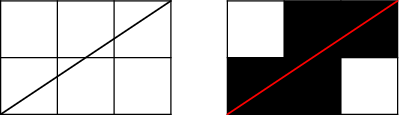# Internet Problem Solving Contest

## Problem R – Rasterized Lines

Tomas is a computer graphics student. He has a homework which is very easy for him. He has to make a program that draws a line from point (0, 0) to (a, b), where integers a, b (a>0, b> 0) are the input of the program.

He uses the following algorithm. He divides the plane into squares 1x1 – these squares are pixels. When the line from (0, 0) to (a, b) intersects a square in more than one point, the square (pixel) will be black. Otherwise it will be white. Look at the example:### Problem specification

Tomas did his homework in 30 minutes and now he is interested in a slightly different problem. Given an integer N, for how many different inputs does his algorithm produce exactly N black pixels?

More precisely, he is only interested in lines beginning in (0, 0) and ending in (a, b), where both a and b are positive integers. Given N, find out how many of these lines will produce exactly N black pixels.

### Input specification

The first line of the input file contains an integer T specifying the number of test cases. Each test case is preceded by a blank line.

Each test case looks as follows: The one and only line contains a positive integer N. You can assume that number N has at most 47 divisors.

### Output specification

For each test case output one line with one integer – the number of lines that use exactly N black pixels.

### Example

Input:

```2

2

6
```

Output:

```3
11
```

In the first test case, the three good lines are those ending in (1,2), (2,1), and in (2,2).

Credits:
Problemsetter(s):lukas
Contest-related materials: lukas, mino